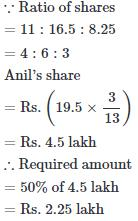# Test: Partnership 2

## 20 Questions MCQ Test Quantitative Techniques for CLAT | Test: Partnership 2

Description
This mock test of Test: Partnership 2 for Quant helps you for every Quant entrance exam. This contains 20 Multiple Choice Questions for Quant Test: Partnership 2 (mcq) to study with solutions a complete question bank. The solved questions answers in this Test: Partnership 2 quiz give you a good mix of easy questions and tough questions. Quant students definitely take this Test: Partnership 2 exercise for a better result in the exam. You can find other Test: Partnership 2 extra questions, long questions & short questions for Quant on EduRev as well by searching above.
QUESTION: 1

### A began a business with Rs. 85,000. He was joined afterwards by B with Rs. 42,500. For how much period does B join, if the profits at the end of the year are divided in the ratio of 3 : 1?

Solution:

Suppose B joined for x months.
Then,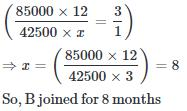QUESTION: 2

### Aman started a business investing Rs. 70,000. Rakhi joined him after six months with an amount of Rs. 1,05,000 and Sagar joined them with Rs. 1.4 lakhs after another six months. The amount of profit earned should be distributed in what ratio among Aman, Rakhi and Sagar respectively, 3 years after Aman started the business?

Solution:

Aman : Rakhi : Sagar
= (70,000 x 36) : (1,05,000 x 30) : (1,40,000 x 24)
= 12 : 15 : 16.

QUESTION: 3

### Arun, Kamal and Vinay invested Rs. 8000, Rs. 4000 and Rs. 8000 respectively in a business. Arun left after six months. If after eight months, there was a gain of Rs. 4005, then what will be the share of Kamal?

Solution: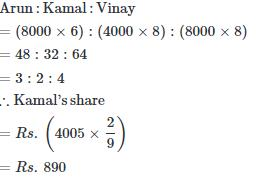QUESTION: 4

Simran started a software business by investing Rs. 50,000. After six months, Nanda joined her with a capital of Rs. 80,000. After 3 years, they earned a profit of Rs. 24,500. What was Simran's share in the profit?

Solution: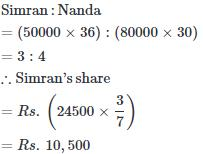QUESTION: 5

Sumit and Ravi started a business by investing Rs 85000 and 15000 respectively. In what ratio the profit earned after 2 years be divided between Sumit and Ravi respectively.

Solution:

Note: If you have clear concept of ratio and proportion chapter then it will really easy for you to solve partnership problems.
P : Q = 85000 : 15000 = 17 : 3
Important to note there that if both have invested for different period of times then we had to multiply with number of months to get the desired ratio.

QUESTION: 6

Rs. 700 is divided among A, B, C so that A receives half as much as B and B half as much as C. Then C's share is

Solution: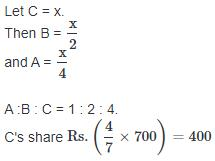QUESTION: 7

Anand and Deepak started a business investing Rs.22,500 and Rs.35,000 respectively. Out of a total profit of Rs. 13,800. Deepak's share is

Solution:

Ratio of their shares = 22500 : 35000
=9 : 14
Deepak's shareQUESTION: 8

A started a business with Rs.21,000 and is joined afterwards by B with Rs.36,000. After how many months did B join if the profits at the end of the year are divided equally?

Solution:

Suppose B joined after x months
then,
21000 × 12 = 36000 × (12 - x)
⇒ 36x = 180
⇒ x = 5

QUESTION: 9

Nirmal and Kapil started a business investing Rs. 9000 and Rs. 12000 respectively. After 6 months, Kapil withdrew half of his investment. If after a year, the total profit was Rs. 4600, what was Kapil’s share initially ?

Solution:

Nirmal : Kapil
= 9000 × 12 : (12000 × 6 + 6000 × 6)
= 1 : 1
Kapils share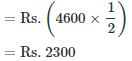QUESTION: 10

Manoj received Rs. 6000 as his share out of the total profit of Rs. 9000 which he and Ramesh earned at the end of one year. If Manoj invested Rs.20000 for 6 months, whereas Ramesh invested his amount for the whole year, what was the amount invested by Ramesh

Solution:

Suppose Ramesh invested Rs. x. Then,
Manoj : Ramesh = 20000 * 6 : x × 12.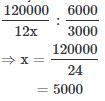QUESTION: 11

Yogesh started a business investing Rs. 45000. After 3 months, Pranab joined him with a capital of Rs. 60000. After another 6 months, Atul joined them with a capital of Rs. 90000. At the end of the year, they made a profit of Rs. 20000. What would be Atuls share in it?

Solution:

Just take care of the months of investment, rest all will be simple.
Yogesh : Pranab : Atul
= 45000 × 12 : 60000 × 9 : 90000 × 3
= 2 : 2 : 1
Atul's share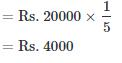QUESTION: 12

Rahul and Bharti are partners in a business. Rahul contributes  1/4th capital for 15 months and Bharti received 2/3 of profit. For how long Bharti money was used.

Solution: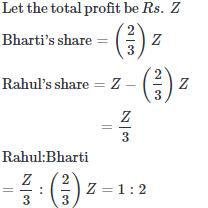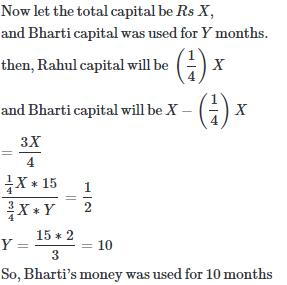QUESTION: 13

P and Q started a business investing Rs 85000 and Rs 15000 resp. In what ratio the profit earned after 2 years be divided between P and Q respectively.

Solution:

In this type of question as time frame for both investors is equal then just get the ratio of their investments.
P : Q = 85000 : 15000
= 85 : 15
= 17 : 3

QUESTION: 14

A, B and C enter into a partnership investing Rs 35000, Rs 45000 and Rs 55000 resp. The respective share of A,B and C in an annual profit of Rs 40500 are.

Solution: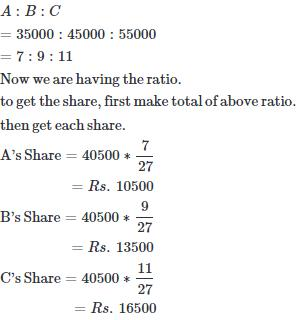QUESTION: 15

Rahul, Arun and Sumit started a business. Rahul invested part, Arun part and rest of the capital was invested by Sumit. The ratio of their profits will be = ?

Solution: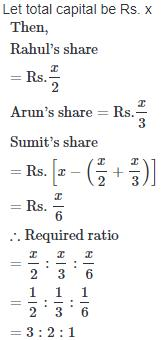QUESTION: 16

Samaira, Mahira and Kiara rented a set of DVDs at a rent of Rs. 578. If they used it for 8 hours, 12 hours and 14 hours respectively, what is Kiara's share of rent to be paid ?

Solution: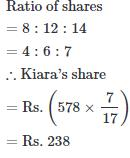QUESTION: 17

P, Q and R invested Rs. 45000, Rs. 70000 and Rs. 90000 respectively to start a business. At the end of 2 years, they earned a profit of Rs.164000. What will be Q's share in the profit ?

Solution: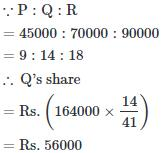QUESTION: 18

A working partner gets 20% as his commission of the profit after his commission is paid. If the working partner's commission is Rs. 8000, then what is the total profit in the business ?

Solution: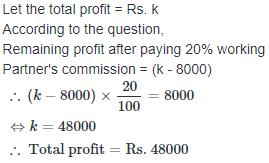QUESTION: 19

We have to divided a sum of Rs. 13950 among three persons A, B and C. B must get the double of A's share and C must get 50 less than the double of B's share. The share of A will be ?

Solution: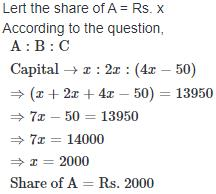QUESTION: 20

Prakash, Sachin and Anil started a business jointly investing Rs. 11 lakh, Rs. 16.5 lakh and Rs.8.25 lakh respectively. The profit earned by them in the business at the end of 3 years was Rs. 5 lakh. What will be 50% of Anil's share in the profit ?

Solution: